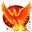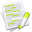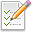#wx.RealPoint¶

A wx.RealPoint is a useful data structure for graphics operations.

It contains floating point x and y members. See wx.Point for an integer version.

Note that the coordinates stored inside a wx.RealPoint object may be negative and that wx.RealPoint functions do not perform any check against negative values.

wx.Point

##Class Hierarchy¶Inheritance diagram for class RealPoint:

##Methods Summary¶

 __init__ Initializes to zero the x and y members. Get Return the point’s properties as a tuple. GetIM Returns an immutable representation of the wx.RealPoint object, based on namedtuple. __bool__ __eq__ __getitem__ __len__ __mul__ __ne__ __nonzero__ __reduce__ __repr__ __setitem__ __str__ __iadd__ __isub__

##Properties Summary¶

 IM x A public C++ attribute of type float. X coordinate of this point. y A public C++ attribute of type float. Y coordinate of this point.

##Class API¶

class wx.RealPoint(object)

Possible constructors:

RealPoint()

RealPoint(x, y)

RealPoint(pt)


A RealPoint is a useful data structure for graphics operations.

### Methods¶

__init__(self, *args, **kw)Overloaded Implementations:

__init__ (self)

Initializes to zero the x and y members.

__init__ (self, x, y)

Initializes the point with the given coordinates.

Parameters
• x (float) –

• y (float) –

__init__ (self, pt)

Converts the given wx.Point (with integer coordinates) to a wx.RealPoint.

Parameters

pt (wx.Point) –

Get(self)

Return the point’s properties as a tuple.

Return type

tuple

Returns

( x, y )

GetIM(self)

Returns an immutable representation of the wx.RealPoint object, based on namedtuple.

This new object is hashable and can be used as a dictionary key, be added to sets, etc. It can be converted back into a real wx.RealPoint with a simple statement like this: obj = wx.RealPoint(imObj).

__bool__(self)

__eq__(self, other)
Return type

bool

__getitem__(self, idx)

__len__(self)

__mul__(self, d)
Return type

wx.RealPoint

__ne__(self, other)
Return type

bool

__nonzero__(self)

__reduce__(self)

__repr__(self)

__setitem__(self, idx, val)

__str__(self)

__iadd__(self, *args, **kw)Overloaded Implementations:

Parameters

sz (wx.Size) –

Parameters

pt (wx.RealPoint) –

__isub__(self, *args, **kw)Overloaded Implementations:

__isub__ (self)

Parameters

sz (wx.Size) –

__isub__ (self)

Parameters

pt (wx.RealPoint) –

### Properties¶

IM

x

A public C++ attribute of type float. X coordinate of this point.

y

A public C++ attribute of type float. Y coordinate of this point.